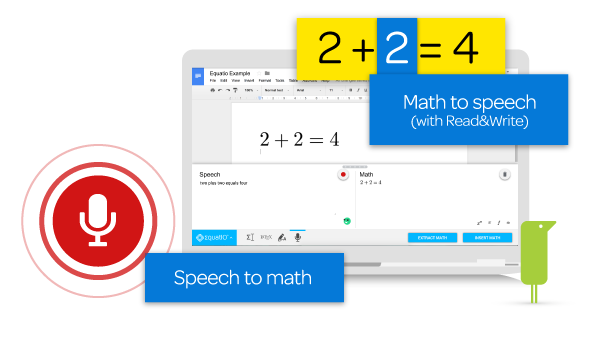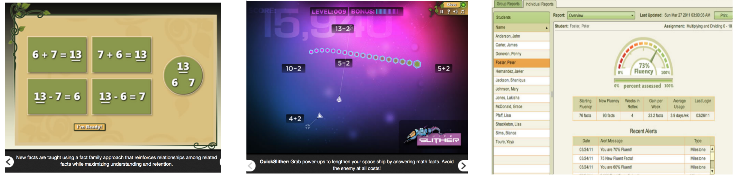####EquatIO – Math Made Digital

Made to help mathematics and STEM teachers and students at all levels, EquatIO® lets everyone create mathematical equations, formulas, Desmos graphs, and more on their computer or Chromebook.

Google Chrome Extension (free) Speech to Text for Math. Also converts handwriting to typed math symbols. Google docs, Chrome, Mac, PC.

Speech is free. Handwriting conversion and math formula prediction is Premium. School group 150 min \$1 per student but Individual costs \$100/yr (!).

####REFLEX MATH